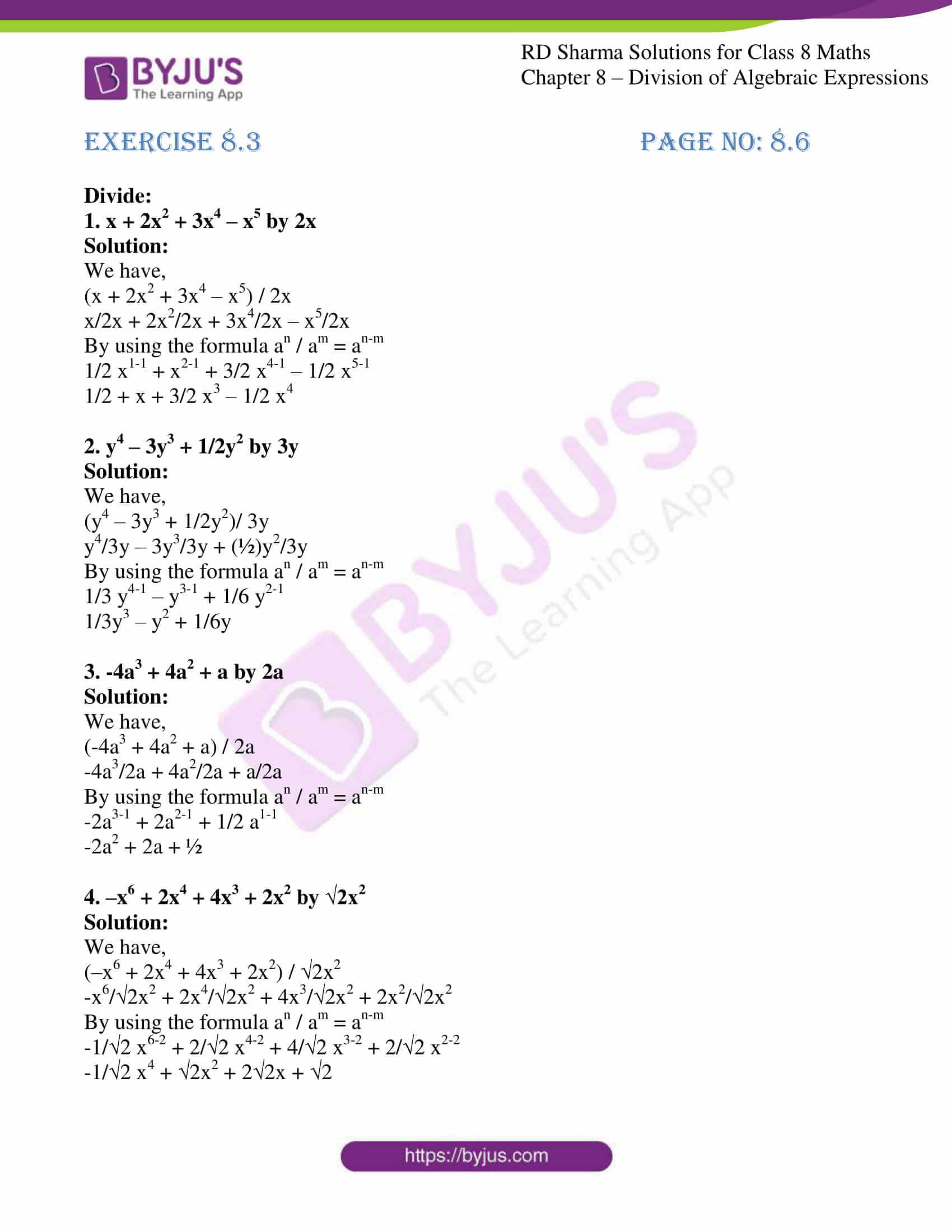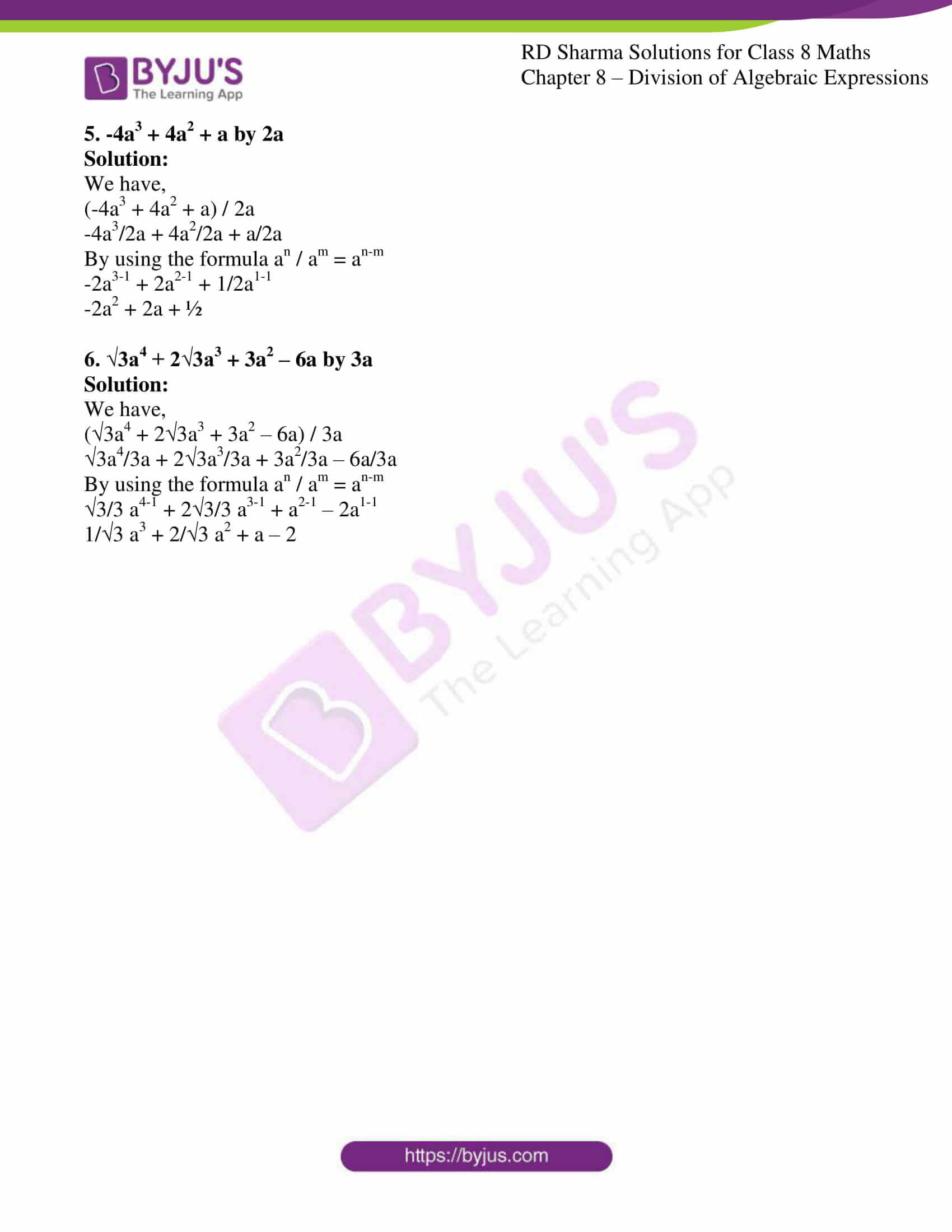# RD Sharma Solutions for Class 8 Maths Chapter 8 Division of Algebraic Expressions Exercise 8.3

RD Sharma Class 8 Maths Chapter 8 Division of Algebraic Expressions are provided here. In Exercise 8.3, we shall discuss the concept of algebraic expressions which are not polynomials and division of polynomials in one variable which includes division of a polynomial by a monomial. The concepts are explained in detail by our experts which helps students prepare for their exams and come out with flying colours in their examination. Students can refer and download the RD Sharma Solutions for Class 8 Maths Exercise 8.3 from the link given below.

## Download the pdf of RD Sharma Solutions for Class 8 Maths Exercise 8.3 Chapter 8 Division of Algebraic Expressions### Access Answers to RD Sharma Solutions for Class 8 Maths Exercise 8.3 Chapter 8 Division of Algebraic Expressions

#### EXERCISE 8.3 PAGE NO: 8.6

Divide:

1. x + 2x2 + 3x4 – x5 by 2x

Solution:

We have,

(x + 2x2 + 3x4 – x5) / 2x

x/2x + 2x2/2x + 3x4/2x – x5/2x

By using the formula an / am = an-m

1/2 x1-1 + x2-1 + 3/2 x4-1 – 1/2 x5-1

1/2 + x + 3/2 x3 – 1/2 x4

2. y4 – 3y3 + 1/2y2 by 3y

Solution:

We have,

(y4 – 3y3 + 1/2y2)/ 3y

y4/3y – 3y3/3y + (½)y2/3y

By using the formula an / am = an-m

1/3 y4-1 – y3-1 + 1/6 y2-1

1/3y3 – y2 + 1/6y

3. -4a3 + 4a2 + a by 2a

Solution:

We have,

(-4a3 + 4a2 + a) / 2a

-4a3/2a + 4a2/2a + a/2a

By using the formula an / am = an-m

-2a3-1 + 2a2-1 + 1/2 a1-1

-2a2 + 2a + ½

4. –x6 + 2x4 + 4x3 + 2x2 by √2x2

Solution:

We have,

(–x6 + 2x4 + 4x3 + 2x2) / √2x2

-x6/√2x2 + 2x4/√2x2 + 4x3/√2x2 + 2x2/√2x2

By using the formula an / am = an-m

-1/√2 x6-2 + 2/√2 x4-2 + 4/√2 x3-2 + 2/√2 x2-2

-1/√2 x4 + √2x2 + 2√2x + √2

5. -4a3 + 4a2 + a by 2a

Solution:

We have,

(-4a3 + 4a2 + a) / 2a

-4a3/2a + 4a2/2a + a/2a

By using the formula an / am = an-m

-2a3-1 + 2a2-1 + 1/2a1-1

-2a2 + 2a + ½

6. √3a4 + 2√3a3 + 3a2 – 6a by 3a

Solution:

We have,

(√3a4 + 2√3a3 + 3a2 – 6a) / 3a

√3a4/3a + 2√3a3/3a + 3a2/3a – 6a/3a

By using the formula an / am = an-m

√3/3 a4-1 + 2√3/3 a3-1 + a2-1 – 2a1-1

1/√3 a3 + 2/√3 a2 + a – 2